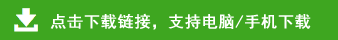# 北师大版三年级数学下册月考检测卷2套（无答案）一、我计算，我聪明
30×4=      400×8=       80×5=     9000×6=     12×3=     42×2=
26×3=     36×2=         18×5=     24×4=       40÷2=     800÷4=
900÷9=    600÷3=       120÷4=     560÷8=     100÷2=    2000÷5=
600×2=     70×7=       600÷2=     70÷7=       210÷3=    320÷8=
40×4=     3500÷7=      160÷8=     26×3=       18×5=     13×8=

26÷1=           46÷2=          69÷3=          84÷4=       66÷3=
55÷5=           270÷9=         3200÷8=       9000÷3=     120÷6=
1000÷2=         400÷8=         3000÷5=       720÷9=     1800÷6=
17÷5=           35÷8=          25÷7=          20÷9=        25÷4=

12＋17×2          100－270÷9           480÷8－25        80×3÷6

60＋240÷3         28＋24×3            2700÷9－250      38×（180÷9）